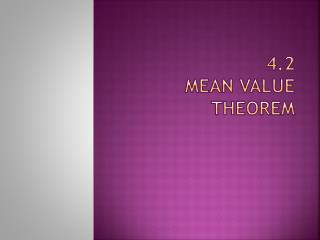Download Presentation4.2 Mean value theorem

# 4.2 Mean value theorem - PowerPoint PPT PresentationDownload Presentation## 4.2 Mean value theorem

- - - - - - - - - - - - - - - - - - - - - - - - - - - E N D - - - - - - - - - - - - - - - - - - - - - - - - - - -
##### Presentation Transcript

1. 4.2Mean value theorem

2. Rolle’s theorem • Exploration: • Sketch a rectangular coordinate plane on a piece of paper. • Label the points (1, 3) and (5, 3). • Draw the graph of a differentiable function that starts at (1, 3) and ends at (5, 3). • WHAT DO YOU NOTICE ABOUT YOUR GRAPH?

3. Rolle’s theorem • If • f is continuous on [a, b], • f is differentiable on (a, b) AND • f(a) = f(b) • Then there is at least one number c in (a, b) such that f’(c) = 0. In other words, if the stipulations above hold true, there is at least one point where the derivative equals 0 (there exists at least one critical point in (a, b) ALSO f has at least an absolute max or min on (a, b)).

4. Rolle’s theorem • Example: Show that f(x) = x4 – 2x2satisfies the stipulations to Rolle’s Theorem on [-2, 2] and find where Rolle’s Theorem holds true. • f is continuous on [-2, 2] • f is differentiable on (-2, 2) • f(-2) = f(2) = 8

5. Mean Value theorem • Consider the graph below: Slope of tangent at c? f’(c) Slope of secant from a to b? a c b

6. Mean value theorem • If • f is continuous at every point on a closed interval [a, b] • f is differentiable at every point on its interior (a, b) • Then there is at least one point c in (a, b) at which In other words, if the stipulations above hold true, there is at least one point where the instantaneous slope equals the average slope.

7. Mean value theorem • Example: a.) Show that the function f(x) = x2 + 2x – 1 on [0, 1] satisfies the Mean Value Theorem. b.) Find each value of c that satisfies the MVT. a.) f is continuous and differentiable on [0, 1] b.) So,

8. Mean value theorem • Example: Given f(x) = 5 – (4/x), find all values of c in the open interval (1, 4) that satisfies the Mean Value Theorem. So,

9. Increasing/decreasing functions Let f be a function defined on an interval I and let x1 and x2 be any two points in I. • fincreases on I if x1 < x2 results in f(x1) < f(x2) • fdecreases on I if x1 > x2 results in f(x1) > f(x2) More importantly relating to derivatives: • Let f be continuous on [a, b] and differentiable on (a, b): • If f’ > 0at each point of (a, b), then fincreases on [a, b] • If f’ < 0 at each point of (a, b), then fdecreases on [a, b] • If f’ = 0 at each point of (a, b), then f is constant on [a, b] (called monotonic)

10. Increasing/decreasing functions • Example: Find the open intervals on which • is increasing or decreasing. 1.) Find critical points

11. Antiderivatives • Find a function F whose derivative is f(x) = 3x2 • F(x) = x3 • A function F is an antiderivative of f on an interval I if F’(x) = f(x) for all x in I. To find an antiderivative of a power function, increase the exponent by 1 and divide the original coefficient by the new exponent. What is the antiderivative of 4x5? (2/3)x6 Look at example 8 on pg. 201!!!